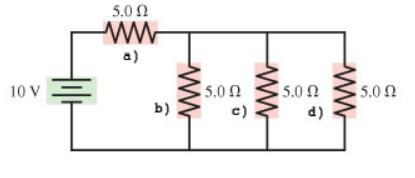# Problem: 1. Find the current through resistor a) in the figure. (Figure 1) Express your answer to two significant figures and include the appropriate units.2. Find the potential difference across resistor a) in the figure.3. Find the current through resistor b) in the figure.4. Find the potential difference across resistor b) in the figure.5. Find the current through resistor c) in the figure.6. Find the potential difference across resistor c) in the figure.7. Find the current through resistor d) in the figure.8. Find the potential difference across resistor d) in the figure 2.

🤓 Based on our data, we think this question is relevant for Professor Gao's class at HUNTER.

###### FREE Expert Solution

Equivalent resistance for resistors in parallel:

$\overline{)\frac{\mathbf{1}}{{\mathbf{R}}_{\mathbf{eq}}}{\mathbf{=}}\frac{\mathbf{1}}{{\mathbf{R}}_{\mathbf{1}}}{\mathbf{+}}\frac{\mathbf{1}}{{\mathbf{R}}_{\mathbf{2}}}{\mathbf{+}}{\mathbf{.}}{\mathbf{.}}{\mathbf{.}}{\mathbf{+}}\frac{\mathbf{1}}{{\mathbf{R}}_{\mathbf{n}}}}$

Equivalent resistance for resistors in series:

$\overline{){{\mathbf{R}}}_{{\mathbf{eq}}}{\mathbf{=}}{{\mathbf{R}}}_{{\mathbf{1}}}{\mathbf{+}}{{\mathbf{R}}}_{{\mathbf{2}}}{\mathbf{+}}{\mathbf{.}}{\mathbf{.}}{\mathbf{.}}{\mathbf{+}}{{\mathbf{R}}}_{{\mathbf{n}}}}$

The potential difference across a resistor:

$\overline{){\mathbf{V}}{\mathbf{=}}{\mathbit{i}}{\mathbf{R}}}$

1.

Resistors b, c, and d are in parallel.

Therefore, 1/Rbcd = 1/5.0 + 1/5.0 + 1/5.0 = 3/5

Rbcd = 1.67Ω###### Problem Details1. Find the current through resistor a) in the figure. (Figure 1)

Express your answer to two significant figures and include the appropriate units.

2. Find the potential difference across resistor a) in the figure.

3. Find the current through resistor b) in the figure.

4. Find the potential difference across resistor b) in the figure.

5. Find the current through resistor c) in the figure.

6. Find the potential difference across resistor c) in the figure.

7. Find the current through resistor d) in the figure.

8. Find the potential difference across resistor d) in the figure 2.